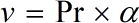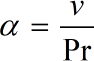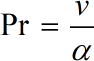Request a Tool

Prandtl Number Calculator

This sophisticated online Prandtl Number Calculator is designed to apply the supplied formula to compute diffusion in flowing systems. Ludwig Prandtl, a German physicist, was the genesis for the name.

Output

Kinematic Viscosity
0

Formula• v = Kinematic Viscosity
• α = Thermal Diffusivity
• Pr = Wetted Perimeter

Defination / Uses

The sign 'v' stands for Kinematic Viscosity. Stokes are generally used to evaluate kinematic viscosity. The viscosity is a measure of the adhesive/cohesive or frictional fluid property and is defined as the fluid resistance to shear or flow. The kinematic formula is used to compute the fluid resistance or density using our advanced online Kinematic Viscosity Calculator.

Output

Thermal Diffusivity
0

Formula• α = Thermal Diffusivity
• Pr = Wetted Perimeter
• v = Kinematic Viscosity

Defination / Uses

A dimensionless number is referred to as the Prandtl Number. The ratio of momentum diffusivity (kinematic viscosity) to thermal diffusivity is known as kinematic viscosity. In a nutshell, the Prandtl Number is the ratio of the thermal conductivity in fluid flow to the product of the coefficient of viscosity and the specific heat under constant pressure.

Prandtl Number
0

Formula• Pr = Wetted Perimeter
• v = Kinematic Viscosity
• α = Thermal Diffusivity

Defination / Uses

A dimensionless number is referred to as the Prandtl Number. The ratio of momentum diffusivity (kinematic viscosity) to thermal diffusivity is known as kinematic viscosity. In a nutshell, the Prandtl Number is the ratio of the thermal conductivity in fluid flow to the product of the coefficient of viscosity and the specific heat under constant pressure.

How to use prandtl number calcultor?

There are only two basic steps.

• First select the option from dropdown. What you need to calculate.
• Then add the other values in their corresponding fields.